# Intermediate Geometry : How to find the length of the side of of an acute / obtuse isosceles triangle

## Example Questions

### Example Question #1 : How To Find The Length Of The Side Of Of An Acute / Obtuse Isosceles Triangle

Find the perimeter of the triangle below.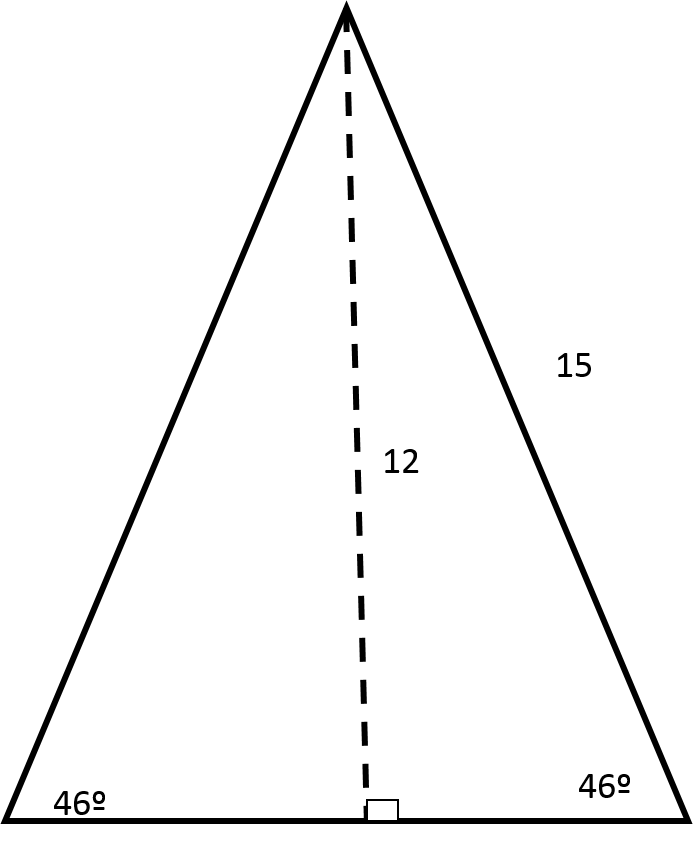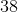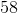Explanation:

Use the Pythagorean Theorem to find the base of the right triangle.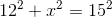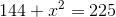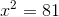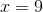Now, because two of the angles in this triangle are the same, this is an isosceles triangle. In an isosceles triangle, the sides that are directly across from the congruent angles are also congruent.

In addition, the height in an isosceles triangle will always cut the 3rd side in half. With this information, fill out the triangle as shown below: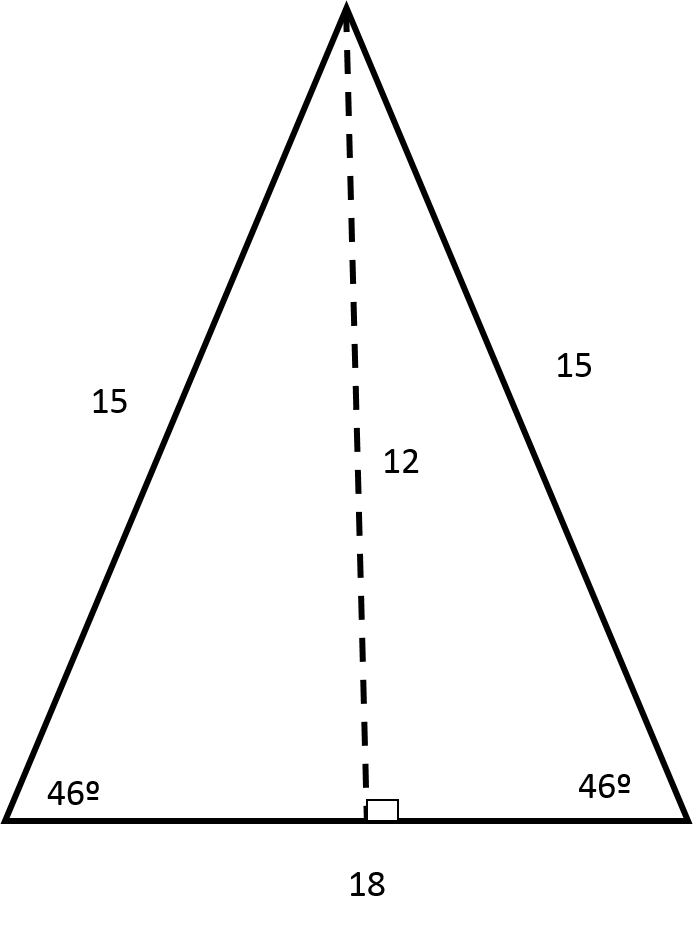To find the perimeter, add up all the sides.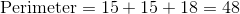### Example Question #2 : How To Find The Length Of The Side Of Of An Acute / Obtuse Isosceles Triangle

Find the perimeter of the triangle below.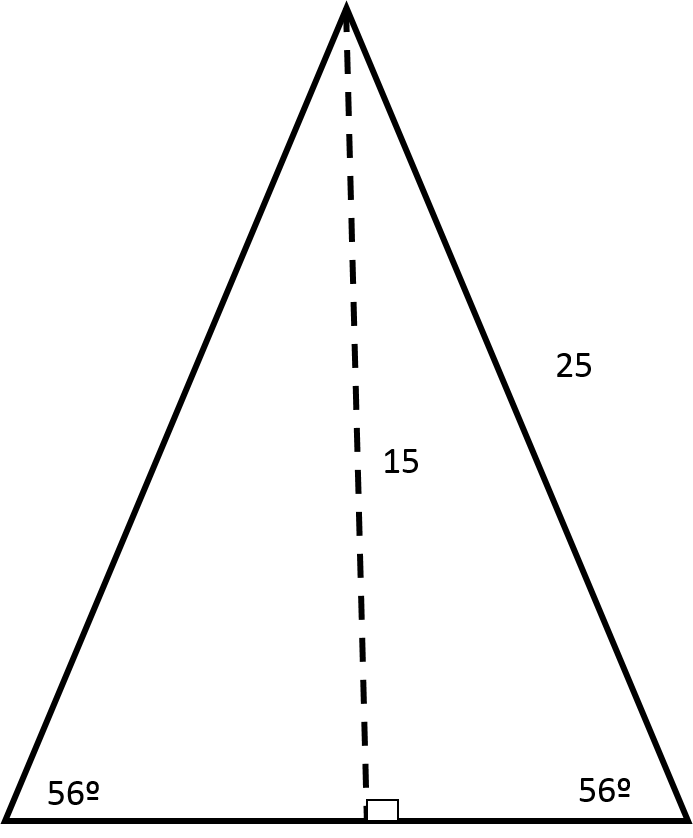Explanation:

Use the Pythagorean Theorem to find the base of the right triangle.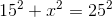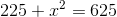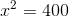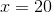Now, because two of the angles in this triangle are the same, this is an isosceles triangle. In an isosceles triangle, the sides that are directly across from the congruent angles are also congruent.

In addition, the height in an isosceles triangle will always cut the 3rd side in half. With this information, fill out the triangle as shown below: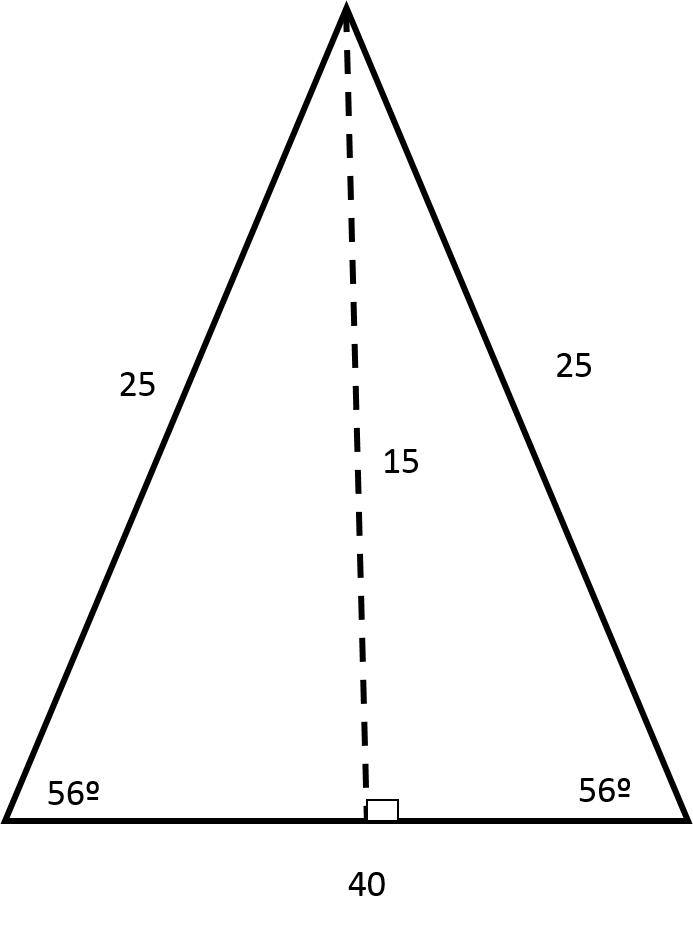To find the perimeter, add up all the sides.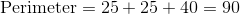### Example Question #3 : How To Find The Length Of The Side Of Of An Acute / Obtuse Isosceles Triangle

A triangle has sides of lengths 14, 18, and 20. Is the triangle scalene or isosceles?

Scalene

Isosceles

Scalene

Explanation:

A triangle with three sides of different length is, by definition, scalene.

### Example Question #4 : How To Find The Length Of The Side Of Of An Acute / Obtuse Isosceles Triangle

Given: Regular Pentagon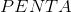with center. Construct segments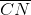and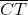to form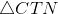.

True or false:is an isosceles triangle.

True

False

True

Explanation:

Below is regular Pentagonwith center, a segment drawn fromto each vertex - that is, each of its radii drawn.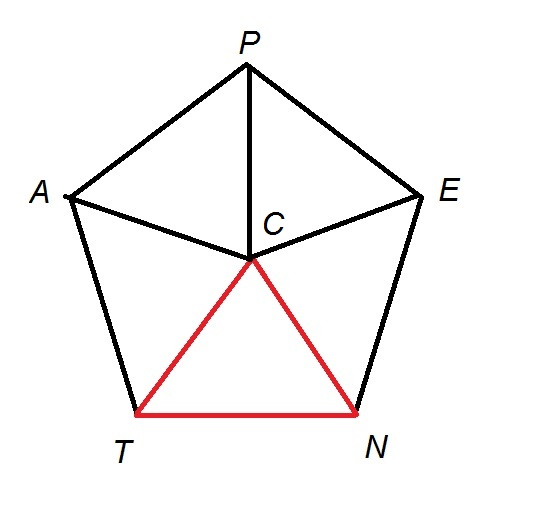By symmetry, all of the radii of a regular pentagon are congruent - specifically,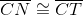. This triangle has at least two congruent sides, so it is isosceles.

### Example Question #5 : How To Find The Length Of The Side Of Of An Acute / Obtuse Isosceles Triangle

If a triangle has side lengths ofand, which of the following can be a length of the third side?Explanation:The triangle inequality theorem states that the sum of the lengths of any two sides must be greater than the length of the third side. The relationship can be represented by the following inequalities: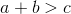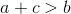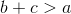The side length ofis the only choice that fits this criteria: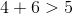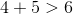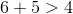### Example Question #6 : How To Find The Length Of The Side Of Of An Acute / Obtuse Isosceles Triangle

If a triangle has side lengths ofand, which of the following could be the length of the third side?Explanation:The triangle inequality theorem states that the sum of the lengths of any two sides must be greater than the length of the third side. The relationship can be represented by the following inequalities:The side length ofis the only choice that fits this criteria: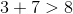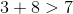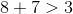### Example Question #7 : How To Find The Length Of The Side Of Of An Acute / Obtuse Isosceles Triangle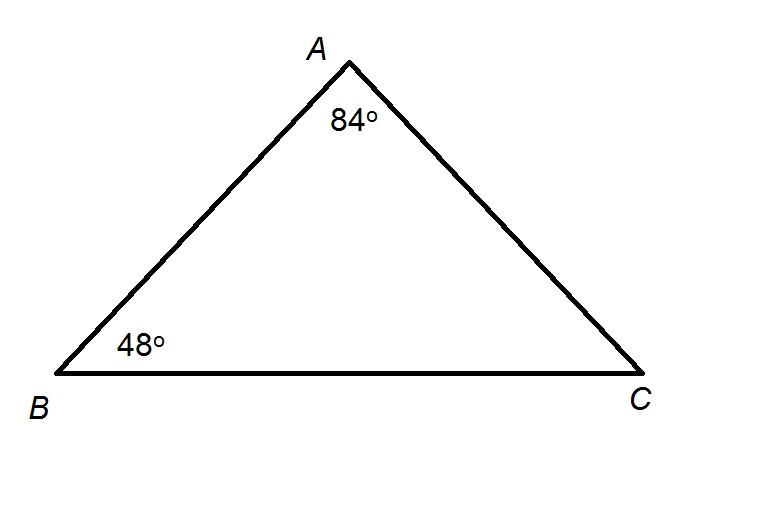Figure NOT drawn to scale.

Refer to the above diagram.

True or false: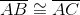.

True

False

True

Explanation:

The sum of the measures of the interior angles of a triangle is, so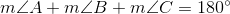Substitute the given two angle measures and solve for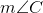: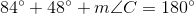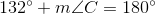Subtract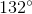from both sides: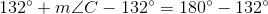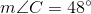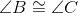, so, by the Converse of the Isosceles Triangle Theorem, their opposite sides are also congruent - that is,### Example Question #8 : How To Find The Length Of The Side Of Of An Acute / Obtuse Isosceles Triangle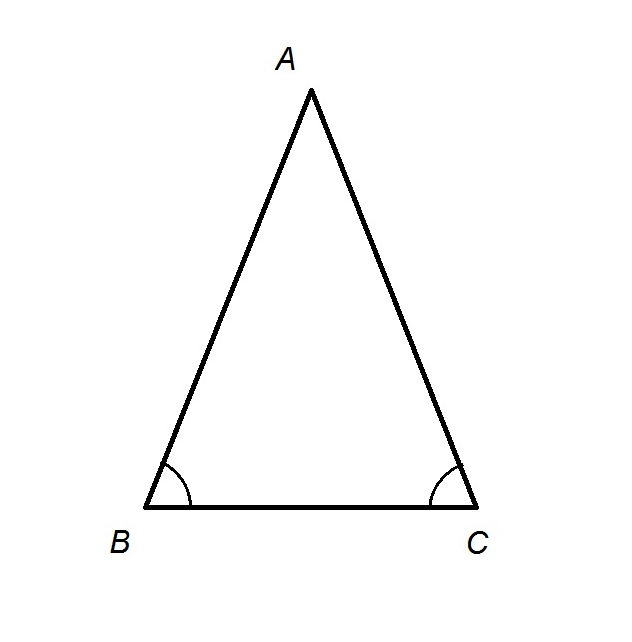Refer to the above triangle. By what statement does it follow that?

The Converse of the Pythagorean Theorem

The Hinge Theorem

The Angle-Side-Angle Postulate

The Converse of the Isosceles Triangle Theorem

The Angle-Angle Postulate

The Converse of the Isosceles Triangle Theorem

Explanation:

We are given that, in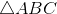, two angles are congruent; specifically,. It is a consequence of the Converse of the Isosceles Triangle Theorem that the sides opposite the angles are also congruent - that is, ..

### Example Question #9 : How To Find The Length Of The Side Of Of An Acute / Obtuse Isosceles Triangle

Given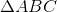such that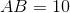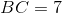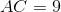, which of the following statements is true?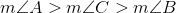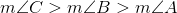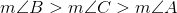It cannot be determined from the information given.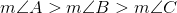Having three sides of different lengths, this triangle is scalene. In any scalene triangle, the angle with greatest measure is opposite the longest side, and the angle with least measure is opposite the shortest side. Therefore, since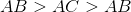, their opposite angles would be in order from greatest to least measure - that is,.